To prove relation reflexive, transitive, symmetric and equivalent

Chapter 1 Class 12 Relation and Functions
Concept wise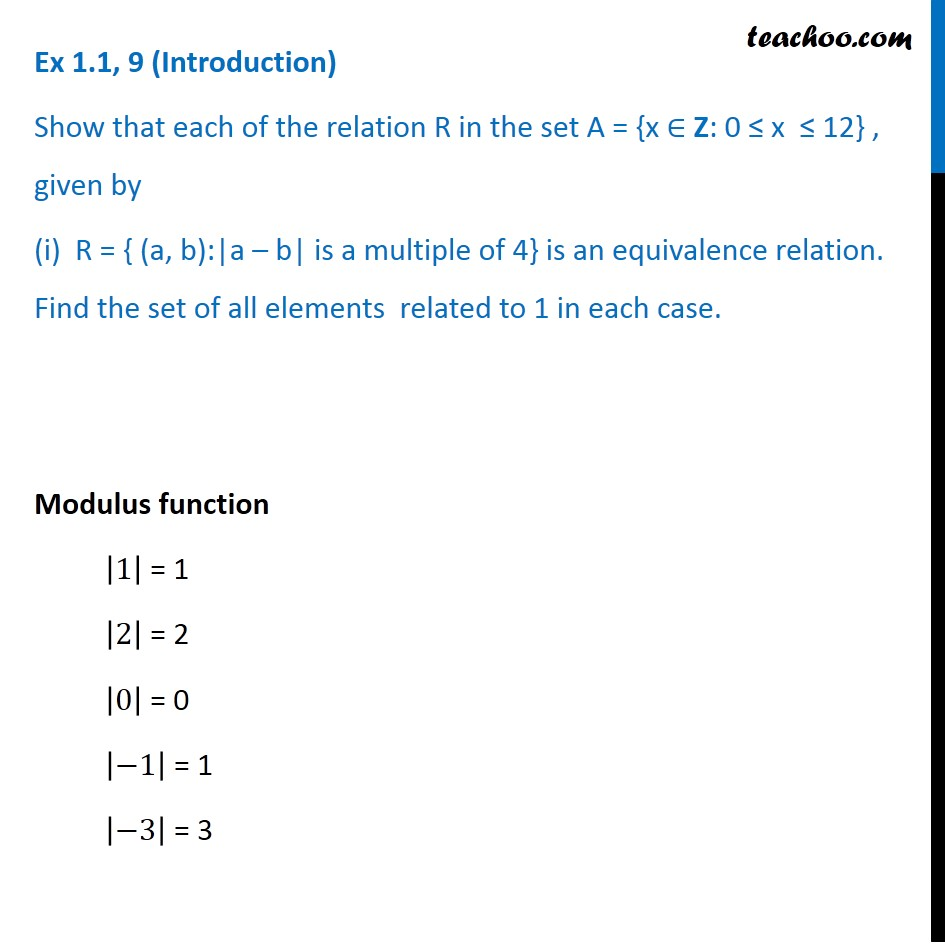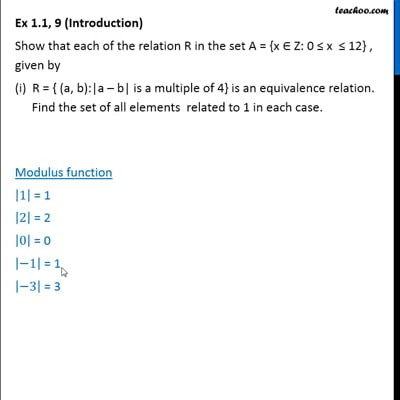This video is only available for Teachoo black users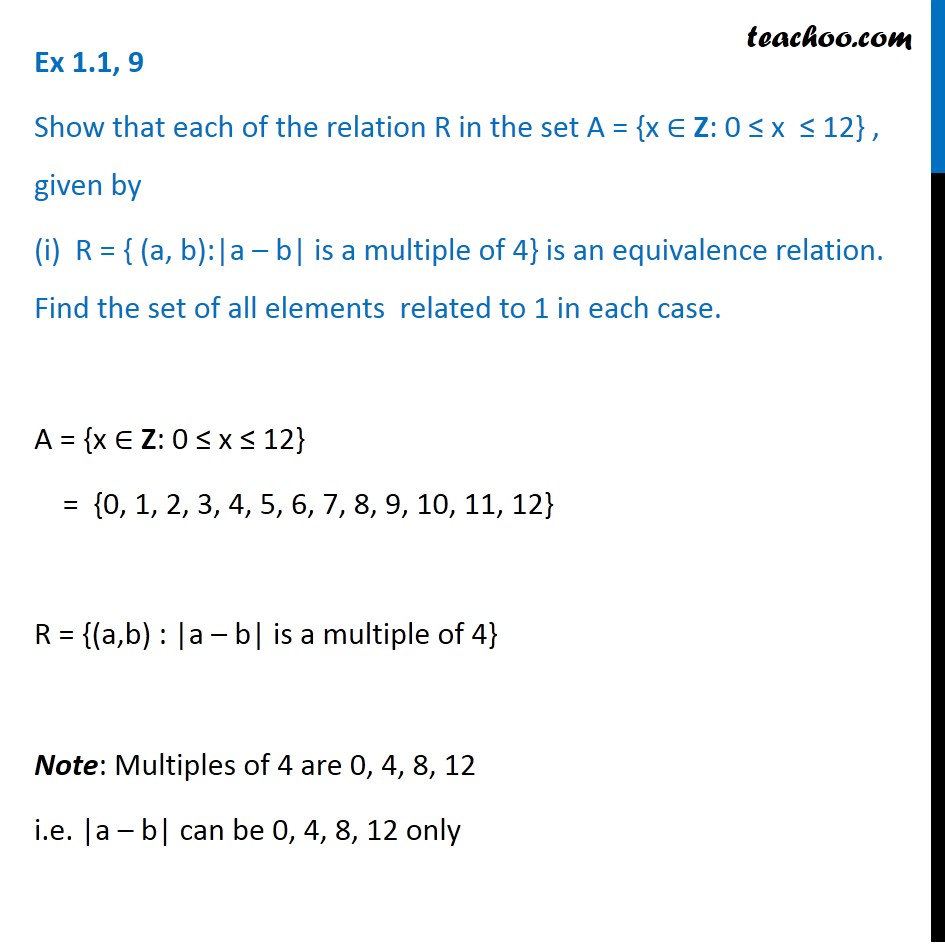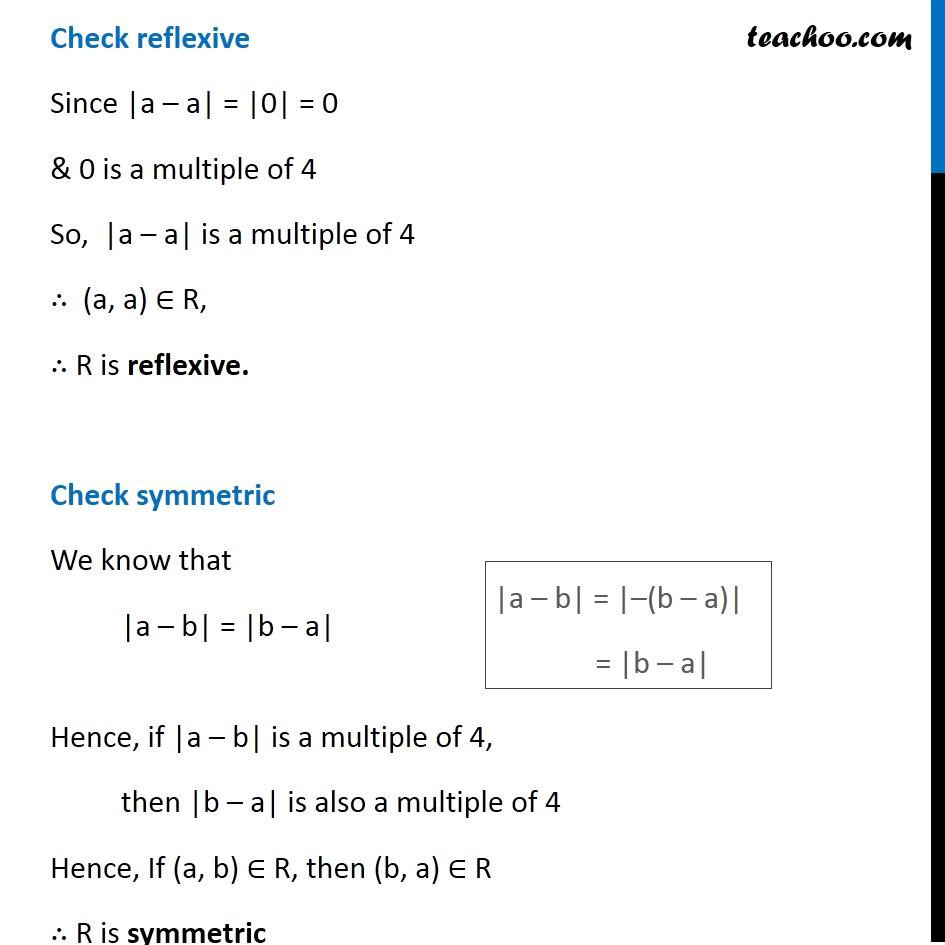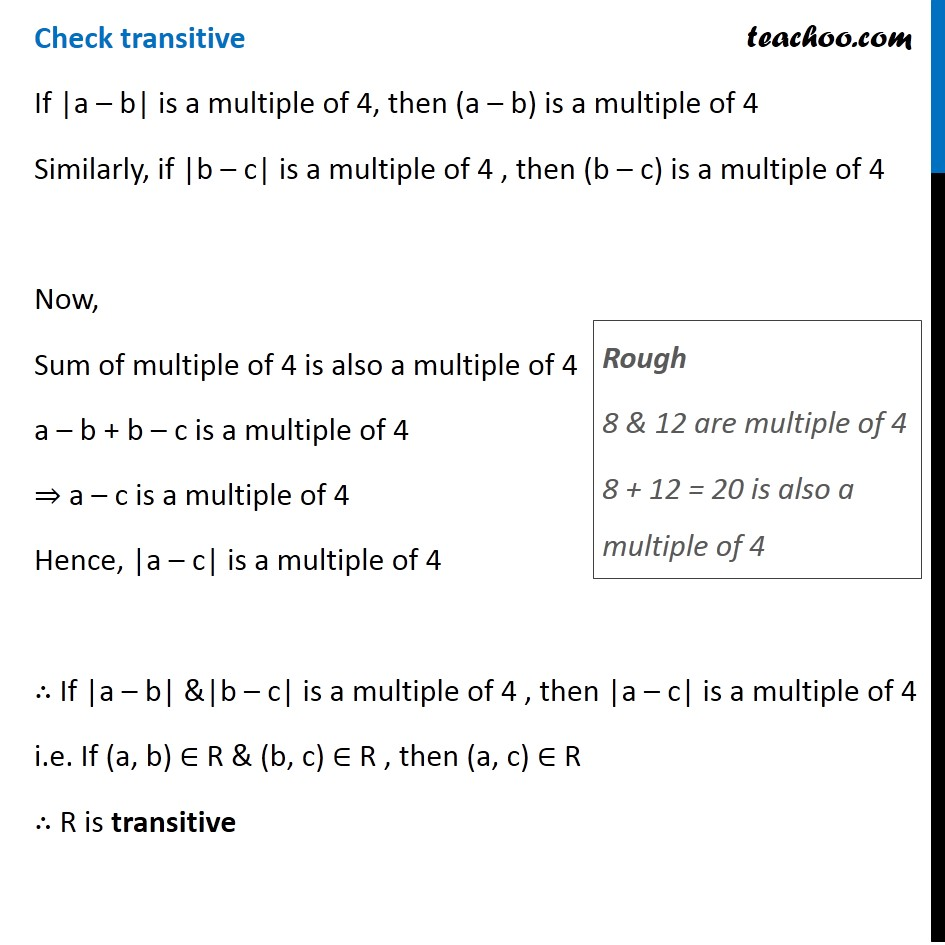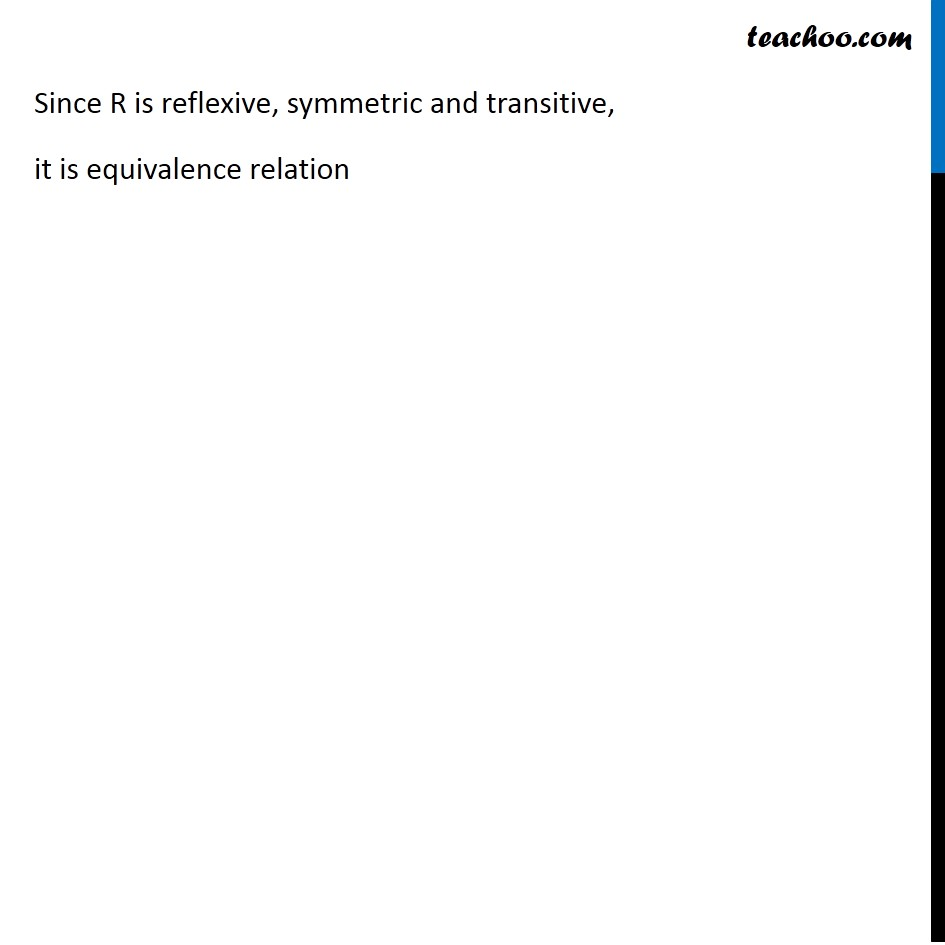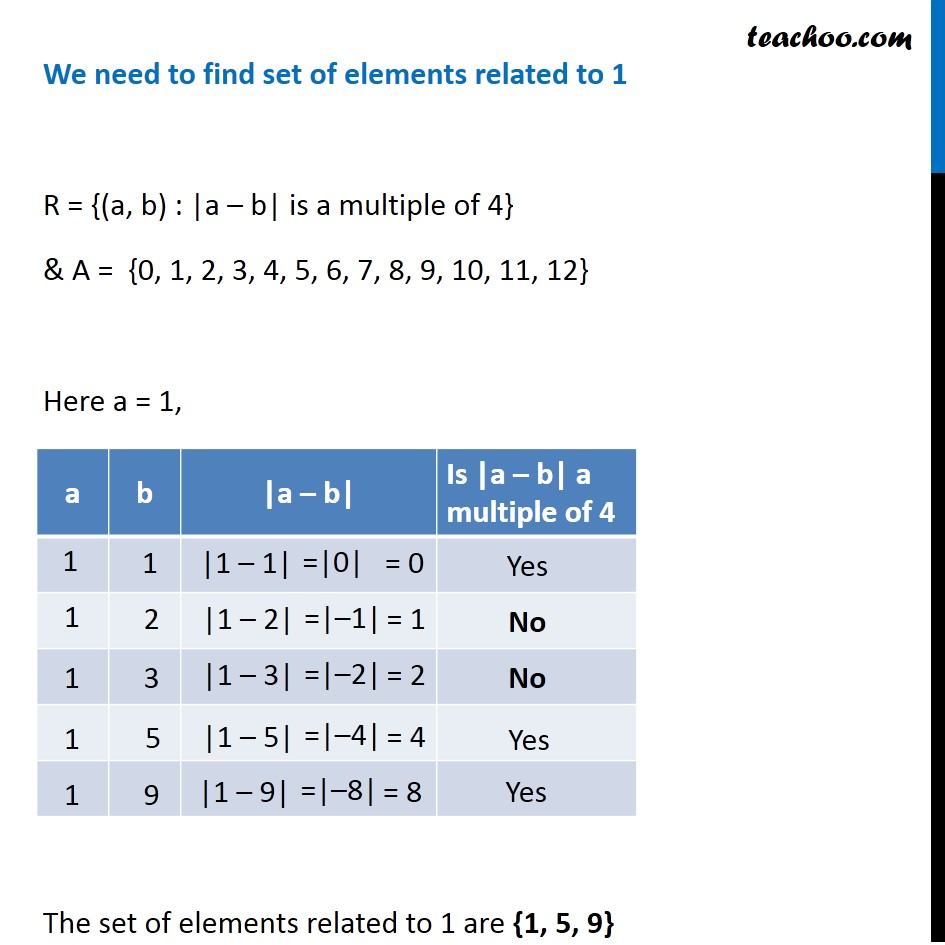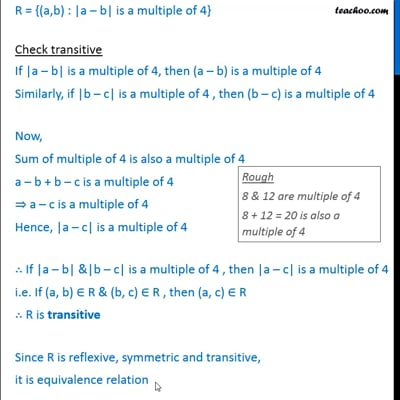This video is only available for Teachoo black users

Introducing your new favourite teacher - Teachoo Black, at only ₹83 per month

### Transcript

Ex 1.1, 9 (Introduction) Show that each of the relation R in the set A = {x ∈ Z: 0 ≤ x ≤ 12} , given by (i) R = { (a, b):|a – b| is a multiple of 4} is an equivalence relation. Find the set of all elements related to 1 in each case. Modulus function |1| = 1 |2| = 2 |0| = 0 |−1| = 1 |−3| = 3 Ex 1.1, 9 Show that each of the relation R in the set A = {x ∈ Z: 0 ≤ x ≤ 12} , given by (i) R = { (a, b):|a – b| is a multiple of 4} is an equivalence relation. Find the set of all elements related to 1 in each case. A = {x ∈ Z: 0 ≤ x ≤ 12} = {0, 1, 2, 3, 4, 5, 6, 7, 8, 9, 10, 11, 12} R = {(a,b) : |a – b| is a multiple of 4} Note: Multiples of 4 are 0, 4, 8, 12 i.e. |a – b| can be 0, 4, 8, 12 only Check reflexive Since |a – a| = |0| = 0 & 0 is a multiple of 4 So, |a – a| is a multiple of 4 ∴ (a, a) ∈ R, ∴ R is reflexive. Check symmetric We know that |a – b| = |b – a| Hence, if |a – b| is a multiple of 4, then |b – a| is also a multiple of 4 Hence, If (a, b) ∈ R, then (b, a) ∈ R ∴ R is symmetric |a – b| = |–(b – a)| = |b – a| Check transitive If |a – b| is a multiple of 4, then (a – b) is a multiple of 4 Similarly, if |b – c| is a multiple of 4 , then (b – c) is a multiple of 4 Now, Sum of multiple of 4 is also a multiple of 4 a – b + b – c is a multiple of 4 ⇒ a – c is a multiple of 4 Hence, |a – c| is a multiple of 4 ∴ If |a – b| &|b – c| is a multiple of 4 , then |a – c| is a multiple of 4 i.e. If (a, b) ∈ R & (b, c) ∈ R , then (a, c) ∈ R ∴ R is transitive Rough 8 & 12 are multiple of 4 8 + 12 = 20 is also a multiple of 4 Since R is reflexive, symmetric and transitive, it is equivalence relation We need to find set of elements related to 1 R = {(a, b) : |a – b| is a multiple of 4} & A = {0, 1, 2, 3, 4, 5, 6, 7, 8, 9, 10, 11, 12} Here a = 1, The set of elements related to 1 are {1, 5, 9}